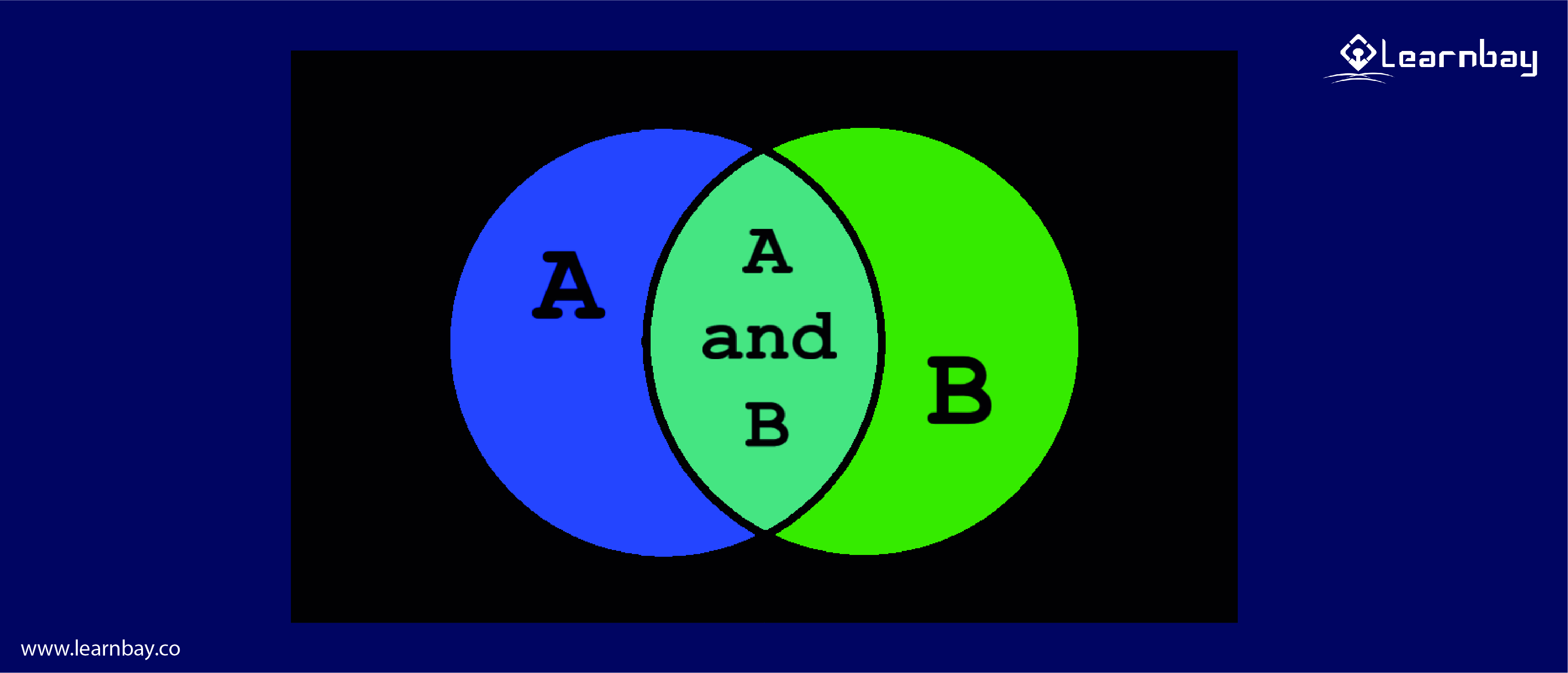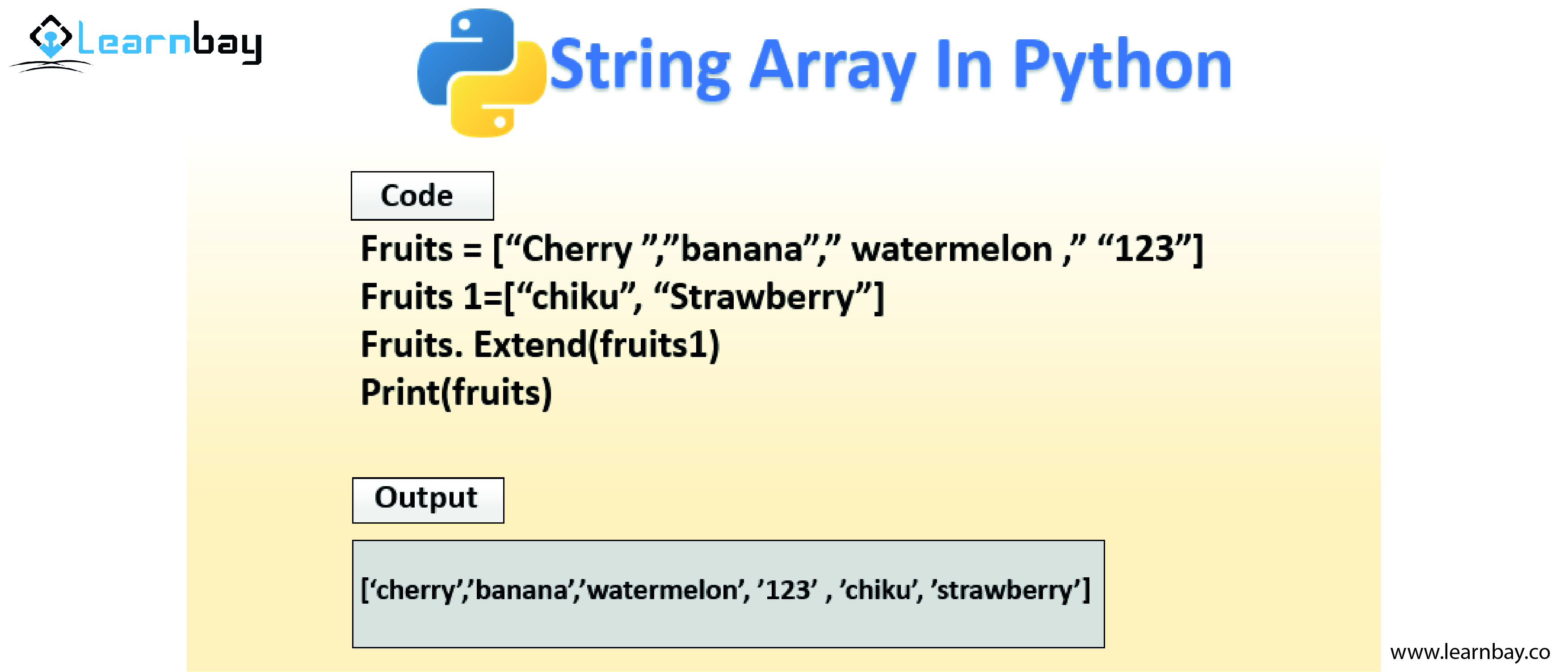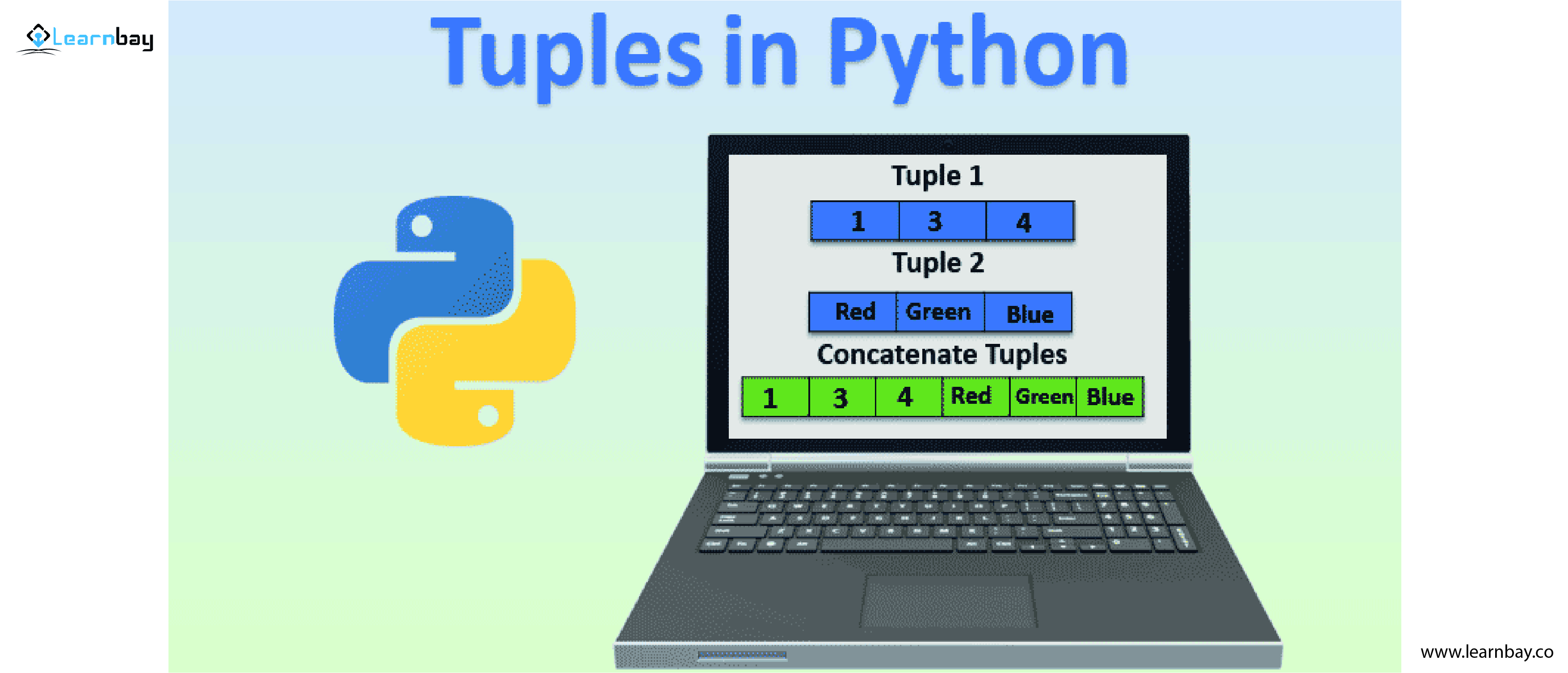# Introduction To Data Types - What Does It Mean In Python Programming?

By Vineeth Kumar Category Data Science Reading time 9-10 mins Published on August 22, 2022

# Introduction To Data Types - What Does It Mean In Python Programming?

When you are working on any programming language, the information has to be written in different forms. This is very important to run the program. Programming is a combination of different data elements, such as integers, values, and strings called data types .

In this article, you will learn about different types of data. Alongside, you will know the process of dealing with various data types and their uses while you are writing a program in python. You will discover their syntax and observe some real-time situations.

## Let us understand what a data type is in python?

The categorization or classification of data elements is called data types.

In a simple definition, It is the value that specifies which operation to perform with the given set of data.

Data types are classes, and variables are instances(objects) for these classes, as everything is an object in python programming.

There are six data types in python

-Numeric

-String

-List

-Tuple

-Set

-DictionaryLet's discuss each data type individually.

# 1. Numeric data type

Numerical data types are shown as numerical values used as integers, float, and complex numbers.

There are four sub-types of numerical data

-Integer

-Float

-Complex

Integer – In this, the value is represented as a whole number without any decimal, irrespective of its positive or negative. The length of the integer is not required to be defined.

Example:

x = 108
y = 142
#As long as the value remains a whole number, it will be the integer.

Float - In this value is represented as a real number with a decimal point irrespective if it is positive or negative. While describing scientific notation, the character's 'e' or 'E' is inserted.

Example:

x = 10.25
y = 12.30

Complex - In this, the value is represented with a combination of a real part and an imaginary part. The imaginary part is denoted with 'j' at the end of the number. Mathematical operations can be expressed with minimal overhead using complex numbers.

Example:

x + yj
where x is the real part and y along with j is the imaginary part# 2. String Data Type in Python

A string is a sequence of Unicode characters. Characters are always enclosed in single, double, or triple quotations to access the values. We use square brackets and indexes to fetch the value from the string. It is represented as 'str' in python.

Example

a = 'Hello Python'
b = "hello python"
c = '''HELLO PYTHON'''

The tasks like slicing, concatenation, and repetition can be performed using string.

Slicing : It slices a particular part of a string.

Example:

a = 'how are you'
print (a[4:11])

Output
are you

Concatenation : It is joining two or more strings together.

Example:

a = 'how '
b = 'are '
c = 'you'
print (a+b+c)

Output
how are you

Repetition : It repeats the same sequence of instructions several times.

Example:

a = 'Hi Python '

print (a*5)

Output

Hi Python Hi Python Hi Python Hi Python Hi Python

# 3.List data Types

A list is represented by placing all the elements inside a square bracket [] and separated by a comma (,). The list can store any data type as items like (int, float, etc.......…). The list is mutable, where we can modify the elements.

Example:

lst = [1, 5, 4.38, 'hello']
print(lst)

Output
[1, 5, 4.38, 'hello']# 4.Tuple data types

A tuple in python is represented by placing all the elements inside a round bracket () and separated by a comma (,). Tuple can store any data type as items like (int, float, etc. ).The only disadvantage of tuples is that it is immutable, where you cannot modify the elements. Since you will not be able to change the item once you have declared it, the operations that you perform on a tuple are less. There is also an advantage, and you can store any values which do not require any change while working on a project.

Example:

tpl = (1, 5, 4.38, 'hello')
print (tpl)

Output
(1, 5, 4.38, 'hello')

# 5. Set data types

A collection of unordered data types is iterable and changeable. It does not have any indexes. It is represented using curly brackets {} and separated by a comma. A set does not contain any duplicate values, so the output will have only distinct values.

Example:

set = {1, 5, 4.38, 'hello'}
print(set)

Output
{1, 'hello', 4.38, 5}

# 6. Dictionary data type

A collection of unordered data values is called a Dictionary. It holds a key-value pair instead of a single-value element. To access an item from a dictionary, refer to the key name by using a key in square brackets. If you want to deal with a high volume of data, a dictionary data type would be most useful. Its function is to revert to which it is optimized. We use curly brackets {} to define this data type.

Example: dictionary = {'Data Science' : 'Python', 'Hi' : 'Hello'}
dictionary['Hi']
dictionary.get('Data Science')

Output
'Hello'
'Python'

# Take away

Now, as you all are aware of different types of data types and we as a programmer feed this food called datatypes to python and execute a reliable output that helps a task to run smoother, faster, and more efficiently. If you are interested in knowing more about python efficiently, you can check out the data science course with special python-support with top-class trainers with best-in-class resources. In case you are just exploring python as a beginner, follow us on Facebook, Youtube, Linkedin, Twitter.